### I. INFLATIONARY BASICS

According to inflationary cosmology [1, 2, 3], the universe expanded exponentially quickly for a fraction of a second very early in its history - growing from a patch as small as 10-26 m, one hundred billion times smaller than a proton, to macroscopic scales on the order of a meter, all within about 10-35 s - before slowing down to the more stately rate of expansion that has characterized the universe's behavior ever since. The driving force behind this dramatic growth, strangely enough, was gravity. [For technical introductions to inflationary cosmology, see [4, 5, 6]; a more popular description may be found in .] Although this might sound like hopeless (or, depending on one's inclinations, interesting) speculation, in fact inflationary cosmology leads to several quantitative predictions about the present behavior of our universe - predictions that are being tested to unprecedented accuracy by a new generation of observational techniques. So far the agreement has been excellent.

How could gravity drive the universal repulsion during inflation? The key to this rapid expansion is that in Einstein's general relativity (physicists' reigning description of gravity), the gravitational field couples both to mass-energy (where mass and energy are interchangeable thanks to Einstein's E = mc2) and to pressure, rather than to mass alone. In the simplest scenario, in which at least the observable portion of our universe can be approximated as being homogeneous and isotropic - that is, having no preferred locations or directions - Einstein's gravitational equations give a particularly simple result. The expansion of the universe may be described by introducing a time-dependent "scale factor," a(t), with the separation between any two objects in the universe being proportional to a(t). Einstein's equations prescribe how this scale factor will evolve over time, t. The rate of acceleration is proportional to the density of mass-energy in the universe,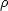, plus three times its pressure, p: d2 a / dt2 = - 4G(+ 3p) a / 3, where G is Newton's gravitational constant (and we use units for which the speed of light c = 1). The minus sign is important: ordinary matter under ordinary circumstances has both positive mass-energy density and positive (or zero) pressure, so that (+3p) > 0. In this case, gravity acts as we would expect it to: All of the matter in the universe tends to attract all of the other matter, causing the expansion of the universe as a whole to slow down.

The crucial idea behind inflation is that matter can behave rather differently from this familiar pattern. Ideas from particle physics suggest that the universe is permeated by scalar fields, such as the Higgs field of the standard model of particle physics, or its more exotic generalizations. (A scalar field takes exactly one value at every point in space and time. For example, one could measure the temperature at every position in a room and repeat the measurements over time, and represent the measurements by a scalar field, T, of temperature. Electric and magnetic fields are vector fields, which carry three distinct components at every point in space and time: the field in the x direction, in the y direction, and in the z direction. Scalar fields are introduced in particle physics to describe certain kinds of particles, just as photons are described in quantum field theories in terms of electromagnetic fields.) These scalar fields can exist in a special state, having a high energy density that cannot be rapidly lowered, such as the arrangement labeled (a) in Fig. 1. Such a state is called a "false vacuum." Particle physicists use the word "vacuum" to denote the state of lowest energy. "False vacua" are only metastable, not the true states of lowest possible energy.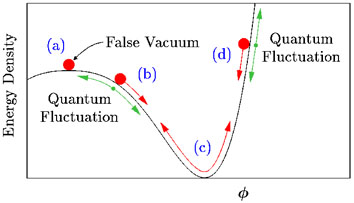Figure 1. In simple inflationary models, the universe at early times is dominated by the potential energy density of a scalar field,. Red arrows show the classical motion of. Whenis near region (a), the energy density will remain nearly constant,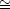f, even as the universe expands. Moreover, cosmic expansion acts like a frictional drag, slowing the motion of: Even near regions (b) and (d),behaves more like a marble moving in a bowl of molasses, slowly creeping down the side of its potential, rather than like a marble sliding down the inside of a polished bowl. During this period of "slow roll,"remains nearly constant. Only afterhas slid most of the way down its potential will it begin to oscillate around its minimum, as in region (c), ending inflation.

In the early universe, a scalar field in such a false vacuum state can dominate all the contributions to the total mass-energy density,. During this period,remains nearly constant, even as the volume of the universe expands rapidly:f = constant. This is quite different from the density of ordinary matter, which decreases when the volume of its container increases. Moreover, the first law of thermodynamics, in the context of general relativity, implies that iff while the universe expands, then the equation of state for this special state of matter must be p-f, a negative pressure. This yields d2 a / dt2 = 8Gf a / 3: Rather than slowing down, the cosmic expansion rate will grow rapidly, driven by the negative pressure created by this special state of matter. Under these circumstances, the scale factor grows as a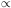eHt, where the Hubble parameter, Ha-1 da / dt, which measures the universe's rate of expansion, assumes the constant value, H[8Gf / 3]1/2. The universe expands exponentially until the scalar field rolls to near the bottom of the hill in the potential energy diagram.

What supplies the energy for this gigantic expansion? The answer, surprisingly, is that no energy is needed . Physicists have known since the 1930s  that the gravitational field carries negative potential energy density. As vast quantities of matter are produced during inflation, a vast amount of negative potential energy materializes in the gravitational field that fills the ever-enlarging region of space. The total energy remains constant, and very small, and possibly exactly equal to zero.

There are now dozens of models that lead to this generic inflationary behavior, featuring an equation of state, p-, during the early universe [4, 9]. This entire family of models, moreover, leads to several main predictions about today's universe. First, our observable universe should be spatially flat. Einstein's general relativity allows for all kinds of curved (or "non-Euclidean") spacetimes. Homogeneous and isotropic spacetimes fall into three classes (Fig. 2), depending on the value of the mass-energy density,. If>c, wherec3H2 / (8G), then Einstein's equations imply that the spacetime will be positively curved, or closed (akin to the two-dimensional surface of a sphere); parallel lines will intersect, and the interior angles of a triangle will always add up to more than 180°. If<c, the spacetime will be negatively curved, or open (akin to the two-dimensional surface of a saddle); parallel lines will diverge and triangles will sum to less than 180°. Only if=c will spacetime be spatially flat (akin to an ordinary two-dimensional flat surface); in this case, all of the usual rules of Euclidean geometry apply. Cosmologists use the letter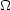to designate the ratio of the actual mass-energy density in the universe to this critical value:/c. Although general relativity allows any value for this ratio, inflation predicts that= 1 within our observable universe to extremely high accuracy. Until recently, uncertainties in the measurement ofallowed any value in the wide range, 0.1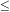2, with many observations seeming to favor0.3. A new generation of detectors, however, has dramatically changed the situation. The latest observations, combining data from the Wilkinson Microwave Anisotropy Probe (WMAP), the Sloan Digital Sky Survey (SDSS), and observations of type Ia supernovae, have measured= 1.012-0.022+0.018  - an amazing match between prediction and observation.Figure 2. According to general relativity, spacetime may be warped or curved, depending on the density of mass-energy. Inflation predicts that our observable universe should be spatially flat to very high accuracy.

In fact, inflation offers a simple explanation for why the universe should be so flat today. In the standard big bang cosmology (without inflation),= 1 is an unstable solution: ifwere ever-so-slightly less than 1 at an early time, then it would rapidly slide toward 0. For example, ifwere 0.9 at 1 s after the big bang, it would be only 10-14 today. Ifwere 1.1 at t = 1 s, then it would have grown so quickly that the universe would have recollapsed just 45 s later. In inflationary models, on the other hand, any original curvature of the early universe would have been stretched out to near-flatness as the universe underwent its rapid expansion (Fig. 3). Quantitatively, |-1|1 / (aH)2, so that while Hconstant and aeHt during the inflationary epoch,gets driven rapidly to 1.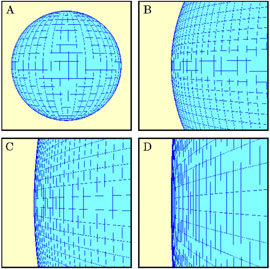Figure 3. (A to D) The expanding sphere illustrates the solution to the flatness problem in inflationary cosmology. As the sphere becomes larger, its surface becomes more and more flat. In the same way, the exponential expansion of spacetime during inflation causes it to become spatially flat.

The second main prediction of inflation is that the presently observed universe should be remarkably smooth and homogeneous on the largest astronomical scales. This, too, has been measured to extraordinary accuracy during the past decades. Starting in the 1960s, Earth-bound, balloon-borne, and now satellite detectors have measured the cosmic microwave background (CMB) radiation, a thermal bath of photons that fills the sky. The photons today have a frequency that corresponds to a temperature of 2.728 K . These photons were released ~ 400,000 years after the inflationary epoch, when the universe had cooled to a low enough temperature that would allow stable (and electrically neutral) atoms to form. Before that time, the ambient temperature of matter in the universe was so high that would-be atoms were broken up by high-energy photons as soon as they formed, so that the photons were effectively trapped, constantly colliding into electrically charged matter. Since stable atoms formed, however, the CMB photons have been streaming freely. Their temperature today is terrifically uniform: After adjusting for the Earth's motion, CMB photons measured from any direction in the sky have the same temperature to one part in 105 .

Without inflation, this large-scale smoothness appears quite puzzling. According to ordinary (noninflationary) big bang cosmology, these photons should never have had a chance to come to thermal equilibrium: The regions in the sky from which they were released would have been about 100 times farther apart than even light could have traveled between the time of the big bang and the time of the photons' release [1, 4, 5, 6]. Much like the flatness problem, inflation provides a simple and generic reason for the observed homogeneity of the CMB: Today's observable universe originated from a much smaller region than that in the noninflationary scenarios. This much-smaller patch could easily have become smooth before inflation began. Inflation would then stretch this small homogeneous region to encompass the entire observable universe.

A third major prediction of inflationary cosmology is that there should be tiny departures from this strict large-scale smoothness, and that these ripples (or "perturbations") should have a characteristic spectrum. Today these ripples can be seen directly as fluctuations in the CMB. Although the ripples are believed to be responsible for the grandest structures of the universe - galaxies, superclusters, and giant voids - in inflationary models they arise from quantum fluctuations, usually important only on atomic scales or smaller. The fieldthat drives inflation, like all quantum fields, undergoes quantum fluctuations in accord with the Heisenberg uncertainty principle. During inflation these quantum fluctuations are stretched proportionally to a(t), rapidly growing to macroscopic scales. The result: a set of nearly scale-invariant perturbations extending over a huge range of wavelengths . Cosmologists parameterize the spectrum of primordial perturbations by a spectral index, ns. A scale-invariant spectrum would have ns = 1.00; inflationary models generically predict ns = 1 to within ~ 10%. The latest measurements of these perturbations by WMAP and SDSS reveal ns = 0.977-0.025+0.039 .

Until recently, astronomers were aware of several cosmological models that were consistent with the known data: an open universe, with0.3; an inflationary universe with considerable dark energy (); an inflationary universe without; and a universe in which the primordial perturbations arose from topological defects such as cosmic strings. Dark energy  is a form of matter with negative pressure that is currently believed to contribute more than 70% of the total energy of the observed universe. Cosmic strings are long, narrow filaments hypothesized to be scattered throughout space, remnants of a symmetry-breaking phase transition in the early universe [15, 16]. [Cosmic strings are topologically nontrivial configurations of fields which should not be confused with the fundamental strings of superstring theory. The latter are usually believed to have lengths on the order of 10-35 m, although for some compactifications these strings might also have astronomical lengths .] Each of these models leads to a distinctive pattern of resonant oscillations in the early universe, which can be probed today through its imprint on the CMB. As can be seen in Fig. 4 , three of the models are now definitively ruled out. The full class of inflationary models can make a variety of predictions, but the prediction of the simplest inflationary models with large, shown on the graph, fits the data beautifully.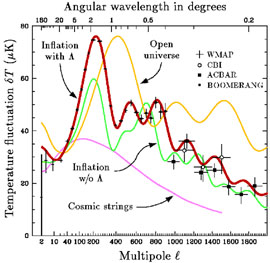Figure 4. Comparison of the latest observational measurements of the temperature fluctuations in the CMB with several theoretical models, as described in the text. The temperature pattern on the sky is expanded in multipoles (i.e., spherical harmonics), and the intensity is plotted as a function of the multipole number. Roughly speaking, each multipolecorresponds to ripples with an angular wavelength of 360° /.